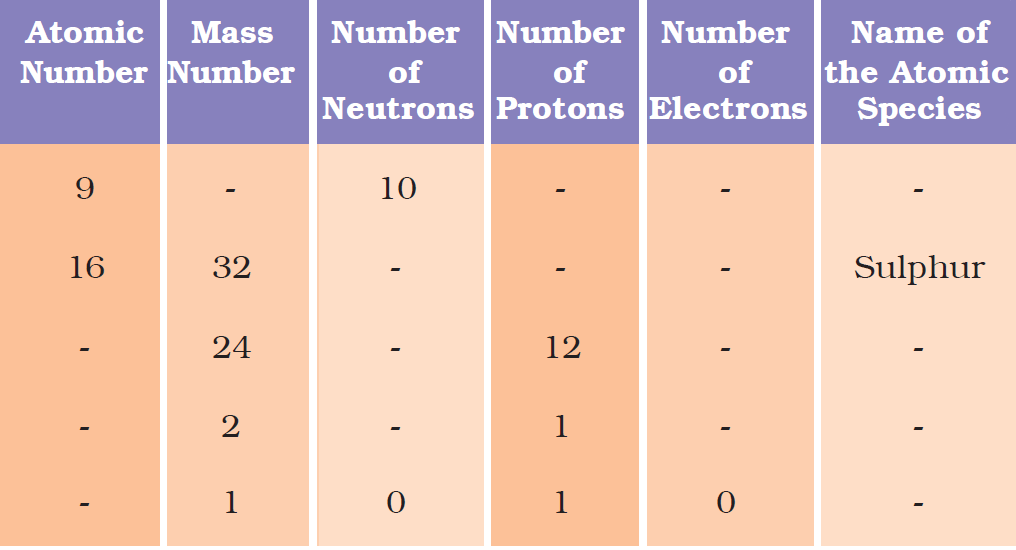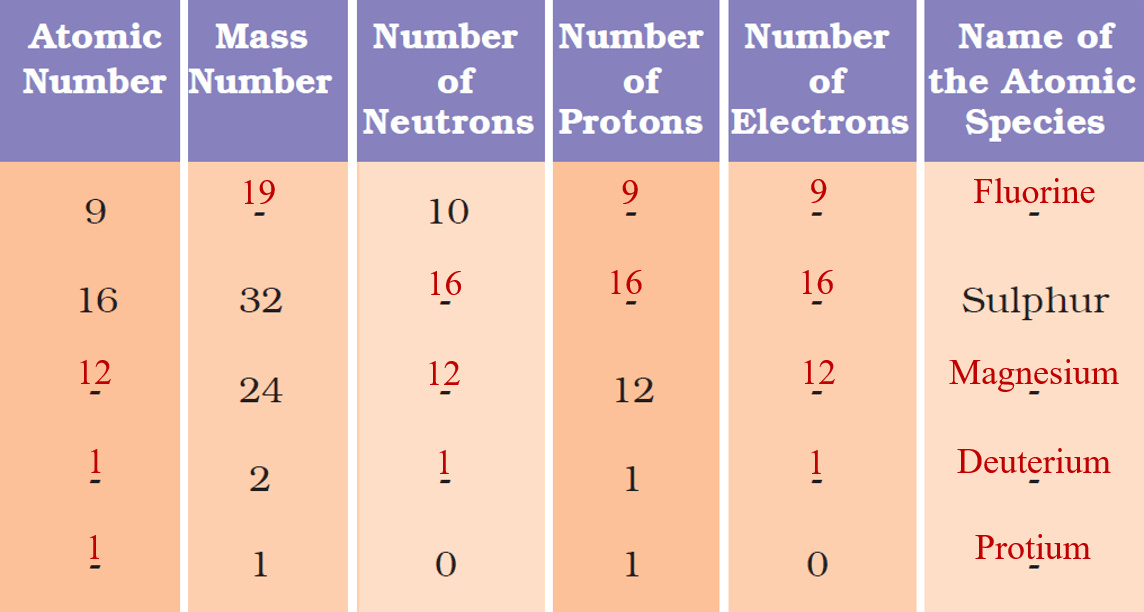# vs.eyeandcontacts.com

## Chapter 4 Structure of the Atom

Intext Questions
Question 1: What are canal rays?

• These are positively charged radiations that consist of particles having charges equal in magnitude.
• Canal rays are also be described as the beam of positive ions obtained by gas-discharge tubes.
• The mass of canal particles is extremely more than that of electrons, about 2000 times larger. These rays are also known as anode rays.

Question 2: If an atom contains one electron and one proton, will it carry any charge or not?
An electron is negatively charged particles (represented by e⁻) and proton is positively charged particle (represented by p⁺). The atom will be neutral because both the electron and proton have same magnitude.

Question 3: On the basis of Thomson’s model of an atom, explain how the atom is neutral as a whole.
An atom consists of a positively charged sphere and the electrons are embedded in it. The negative and positive charges are equal in magnitude. So, the atoms as a whole is electrically neutral.

Question 4: On the basis of Rutherford’s model of an atom, which sub-atomic particle is present in the nucleus of an atom?
On the basis of Rutherford’s model of an atom, the positively charged particles or protons are present in the nucleus of an atom.

Question 5: Draw a sketch of Bohr’s model of an atom with three shells.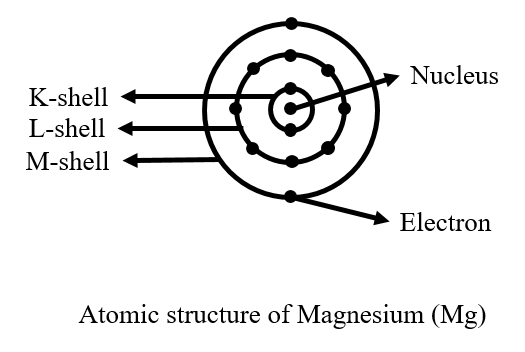Question 6: What do you think would be the observation if the α-particle scattering experiment is carried out using a foil of a metal other than gold?
No, the observation would not be same if the α-particle scattering experiment is carried out using a foil of a metal other than gold. If the foil of heavy metal like platinum (having a very heavy nucleus) is used, the observation in α-particle scattering would be same as that in gold foil experiment.
If the foil of light metal like lithium (having a very light nucleus) is used, then the fast moving heavy α-particles may even push the light nucleus aside and may not be deflected.

Question 7: Name the three sub-atomic particles of an atom.
Three sub-atomic particles of an atom are
• Proton
• Electron
• Neutron

Question 8: Helium atom has an atomic mass of 4 u and two protons in its nucleus. How many neutrons does it have?

Atomic mass of helium atom = 4 u
Number of protons = 2
Number of neutrons = ?
Atomic mass of atom = Number of protons + Number of neutrons
4 = 2 + N
N = 2
Therefore, the number of neutrons present in helium atom is 2.

Question 9: Write the distribution of electrons in carbon and sodium atoms.

Carbon atom has total of 6 electrons.
Electronic distribution = 2, 4
Electrons in K-shell = 2
Electrons in L-shell = 4

Sodium atom has total of 11 electrons
Electronic distribution = 2, 8, 1
Electrons in K-shell = 2
Electrons in L-shell = 8
Electrons in M-shell = 1

Question 10: If K and L shell of an atom are full, then what would be the total number of electrons in the atom?
K-shell can hold 2 electrons. L-shell can hold 8 electrons.
Total number of electrons in atom = Number of electrons in K-shell + Number of electrons in L-shell = 2 + 8 = 10 electrons.

Question 11: How will you find the valency of chlorine, sulphur, and magnesium?
The number of electrons gained, lost or shared so as to fill the complete shell, gives us directly the combining capacity of the element, that is, valency.
If the number of electrons in the outermost shell of an atom is close to its full capacity, valency is determined in different way. The chlorine atom has 7 electrons in outermost shell. The valency could be 7. But it is easier for chlorine to gain 1 electron instead of losing 7 electrons. So, its valency is determined by subtracting seven electrons from the octet configuration and this gives 1 as the valency for chlorine.
Similarly in sulphur atom the outermost shell has 6 electrons. It is easier for sulphur to gain 2 electrons rather than losing 6 electrons. So, its valency is determined by subtracting 6 electrons from the octet configuration and this gives 2 as the valency for sulphur.
In magnesium, the outermost shell has 2 electrons. Instead of gaining 6 electrons it is easier for magnesium to lose 2 electrons. So, its valency becomes 2.

Question 12: If the number of electrons in an atom is 8 and number of protons is also 8, then
i) what is the atomic number of the atom?
ii) what is the charge on the atom?
The atomic number of any atom is represented by letter ‘Z’. All atoms of an element have the same atomic number. Atomic number is the number of protons of an atom. Since, the number of protons in given atom is 8, the atomic number is also 8. Since, the total number of protons and electrons are equal, the charge on the atom is said to be neutral.

Question 13: With the help of Table 4.1, find out the mass number of oxygen and sulphur atom.
Mass number of any atom is equal to the sum of the number of protons and neutrons in the nucleus.
The mass number of oxygen = number of protons in oxygen atom + number of neutrons in oxygen atom = 8 + 8 = 16 u.
The mass number of sulphur = number of protons in sulphur atom + number of neutrons in sulphur atom = 16 + 16 = 32 u.

Question 14: For the symbol H, D and T tabulate three sub-atomic particles found in each of them.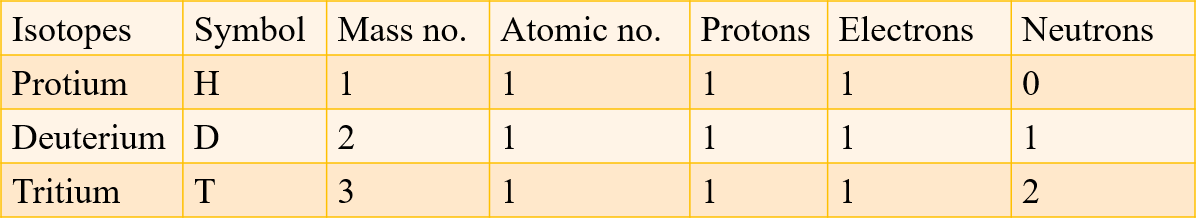Question 15: Write the electronic configuration of any one pair of isotopes and isobars.
Isotopes are defined as the atoms of the same element having same atomic number but different mass number. Example: Protium (¹H₁), Deuterium (²H₁), Tritium (³H₁)
Electronic configuration: K-shell: 1
Isobars are defined as the atoms of different elements having different atomic numbers but same mass number. Example: Calcium (⁴⁰Ca₂₀), Argon (⁴⁰Ar₁₈)
Electronic configuration of ⁴⁰Ca₂₀: K-shell:2; L-shell:8, M-shell:8; N-shell:2
Electronic configuration of ⁴⁰Ar₁₈: K-shell:2; L-shell:8, M-shell:8

Exercise Questions
Question 1: Compare the properties of electrons, protons and neutrons.

• Protons
→ Positively charged particle in atom
→ Located in the nucleus
→ Mass is 1 amu
→ Attracted towards negatively charged particles

• Electrons
→ Negatively charged particle in atom
→ Located outside the nucleus
→ Mass is negligible
→ Attracted towards positively charged particles

• Neutrons
→ No charge
→ Located in the nucleus
→ Mass is 1 amu
→ Don’t get attracted to any charged particles

Question 2: What are the limitations of JJ Thomson’s model of the atom?
The model failed to explain the outcome of alpha-scattering particle, which was conducted by Rutherford. The model failed to explain why the majority of these alpha-particles pass through gold foil, while some diverted at small or big angle while a few rebounded completely, returning on their path. It did not provide any experimental evidence and was established on imagination.

Question 3: What are the limitations of Rutherford’s model of an atom?
The revolution of the electrons in a circular orbit is not expected to be stable. Any particle in a circular orbit would undergo acceleration. During acceleration, charged particles would radiate energy. Thus, the revolving electron would lose energy and finally fall into the nucleus. If this were so, the atom should be highly unstable and hence matter would not exist in the form that we know. Atoms are quite stable.

Question 4: Describe Bohr’s model of the atom.
Atom consist of heavy positively charged nucleus. Mass of atom is concentrated in nucleus. The electrons in an atom revolve around the nucleus in definite circular paths called orbits or energy level.

Question 5: Compare all the proposed models of an atom given in this chapter.

• Thomson’s model
→ Sphere is positively charged.
→ Electrons are negatively charged and scattered all through the inside of the sphere.
→ Positively charged and Negatively charged particles are equal in magnitude.
→ The net charge in the atom is zero.

• Rutherford’s model
→ Nucleus is at the centre and is positively charged, holding the entire mass.
→ Electrons are negatively charged, revolving in a well-defined circular path.
→ In comparison with the nucleus, the size of the atom is very large.
→ Force of attraction of the electrons towards the nucleus is balanced. As a result, electrons are not drawn close to the nucleus.

• Bohr
→ Nucleus is present at the centre and is positively charged.
→ Mass of the atom resides in the nucleus.
→ Electrons are negatively charged, revolving around but do not radiate energy (i.e. move in discrete orbits).
→ The distinct orbits are K, L, M, and N.

Question 6: Summarize the rules for writing the distribution of electrons in various shells for the first eighteen elements.
The following rules are followed for writing the number of electrons in different energy levels or shells:
• The maximum number of electrons present in a shell is given by the formula 2n², where ‘n’ is the orbit number or energy level index, 1,2,3,… Hence the maximum number of electrons in different shells are as follows: first orbit or K-shell will be = 2 × 12 = 2, second orbit or L-shell will be = 2 × 22 = 8, third orbit or M-shell will be = 2 × 32 = 18, fourth orbit or N-shell will be = 2 × 42 = 32, and so on.
• The maximum number of electrons that can be accommodated in the outermost orbit is 8.
• Electrons are not accommodated in a given shell, unless the inner shells are filled. That is, the shells are filled in a step-wise manner.

Question 7: Define valency by giving the examples of silicon and oxygen.
The definite combining capacity of the atoms are called valency. To measure valency, we can figure out the number of electrons that are required to complete shell in which it is contained or losing excess electrons, if present, once the filling is complete. Example:
• Silicon
The atomic number of silicon is 14. The number of electrons is equal to the number of protons in silicon i.e. 14. The distribution of electrons in silicon atom is K-2, L-8, M-4.
So, to fill the M-shell, 4 electrons are required. Therefore, its valency is 8-4 = 4.
• Oxygen
The atomic number of oxygen is 8. The number of electrons is equal to the number of protons in oxygen i.e. 8. The distribution of electrons in silicon atom is K-2, L-6.
So, to fill the L-shell, 2 electrons are required. Therefore, its valency is 8-6 = 2.

Question 8: Explain with examples:
i) Atomic number
ii) Mass number
iii) Isotope
iv) Isobar
Give any two uses of isotopes.

i) The number of protons of an atom determines its atomic number. It is represented by ‘Z’. Example: H = 1, C = 6 etc.
ii) It is defined as the sum of the total protons and neutrons present in the nucleus of atom. Example: There are 2 protons and neutrons in helium atom. So, its mass is 4 u.
iii) Isotopes are defined as the atoms of the same element have same atomic number but different mass number. Example: Protium, Deuterium and Tritium have the same atomic number but different mass number.
iv) Isobars are defined as the atoms of the different element having different atomic number but same mass number. Example: Argon and Calcium have different atomic number but same mass number.

Use of isotopes
• An isotope of uranium is used as a fuel in nuclear reactors.
• An isotope of cobalt is used in the treatment of cancer.

Question 9: Na⁺ has completely filled K and L shells. Explain.
The atomic number of sodium is 11. So, neutral sodium atom has 11 electrons. Na⁺ has 10 electrons (as it gives away 1 electron). Out of 10, K shell can hold 2 electrons and L shell can hold 8 electrons. Hence, Na⁺ has completely filled K and L shells.

Question 10: If bromine atom is available in the form of, say, two isotopes ⁷⁹Br₃₅ (49.7%) and ⁸¹Br₃₅ (50.3%), calculate the average atomic mass of bromine atom.

Isotope of bromine with atomic mass 79 u = 49.7%
Contribution of ⁷⁹Br₃₅ to atomic mass of Br = 79 × 49.7/100 = 39.26 u
Isotope of bromine with atomic mass 81 u = 50.3%
Contribution of ⁸¹Br₃₅ to atomic mass of Br = 81 × 50.3/100 = 40.74 u
The average atomic mass of bromine atom is 39.26 + 40.74 = 80 u.

Question 11: The average atomic mass of a sample of an element X is 16.2 u. What are the percentages of isotopes ¹⁶X₈ and ¹⁸X₈ in the sample?
Let the percentage of ¹⁶X₈ be ‘a’ and ‘100 - a’ of ¹⁸X₈.
16.2 = 16a/100 + 18(100 - a)/100
1620 = 16a + 1800 - 18a
180 = 2a
a = 90%

Percentage of isotope ¹⁶X₈ in sample = 90%
Percentage of isotope ¹⁸X₈ in sample = 100 - 90 = 10%

Question 12: If Z = 3, what would be the valency of the element? Also name the element.

Z of atom (atomic number) = 3
Valency of element = ?
The electronic configuration of element = 2, 1 (K-2, L-1)
The element can lose its one electron, hence its valency is 1.
The element with atomic number 3 is lithium.

Question 13: Composition of the nuclei of two atomic species X and Y are given under as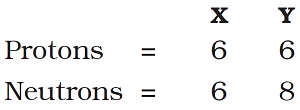Give the mass number of X and Y. What is the relation between two species?

Mass number of X = number of protons + number of neutrons
= 6 + 6 = 12 u
Mass number of Y = number of protons + number of neutrons
= 6 + 8 = 14 u
The atomic number of X and Y is 6 (the number of protons determine the atomic number). The mass number of X and Y are 12 u and 14 u respectively. So, the relationship between X and Y is that they are isotopes (i.e. having same atomic number but different mass number).

Question 14: For the following statements, write T for True and F for False.
a) J.J. Thomson proposed that the nucleus of an atom contains only nucleons.
b) A neutron is formed by an electron and a proton combining together. Therefore, it is neutral.
c) The mass of an electron is about 1/2000 times that of proton.
d) An isotope of iodine is used for making tincture iodine, which is used as a medicine.

a) False
b) False
c) True
d) False

Question 15: Put tick (✔) against correct choice and cross (×) against wrong choice in questions 15, 16 and 17
Question 15:  Rutherford’s alpha-particle scattering experiment was responsible for the discovery of
a) Atomic Nucleus
b) Electron
c) Proton
d) Neutron
a) Atomic Nucleus

Question 16: Isotopes of an element have
a) the same physical properties
b) different chemical properties
c) different number of neutrons
d) different atomic numbers.
c) different number of neutrons

Question 17: Number of valence electrons in Cl⁻ ion are:
a) 16
b) 8
c) 17
d) 18
b) 8

Question 18: Which one of the following is a correct electronic configuration of sodium?
a) 2,8
b) 8,2,1
c) 2,1,8
d) 2,8,1
d) 2,8,1

Question 19: Complete the following table: